Courses

# Chemistry Test 10 - States Of Matter, Alkyl Halide And Aryl Halide

## 25 Questions MCQ Test Mock Test Series for JEE Main & Advanced 2022 | Chemistry Test 10 - States Of Matter, Alkyl Halide And Aryl Halide

Description
This mock test of Chemistry Test 10 - States Of Matter, Alkyl Halide And Aryl Halide for JEE helps you for every JEE entrance exam. This contains 25 Multiple Choice Questions for JEE Chemistry Test 10 - States Of Matter, Alkyl Halide And Aryl Halide (mcq) to study with solutions a complete question bank. The solved questions answers in this Chemistry Test 10 - States Of Matter, Alkyl Halide And Aryl Halide quiz give you a good mix of easy questions and tough questions. JEE students definitely take this Chemistry Test 10 - States Of Matter, Alkyl Halide And Aryl Halide exercise for a better result in the exam. You can find other Chemistry Test 10 - States Of Matter, Alkyl Halide And Aryl Halide extra questions, long questions & short questions for JEE on EduRev as well by searching above.
QUESTION: 1

Solution:
QUESTION: 2

Solution:
QUESTION: 3

### In reaction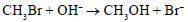proceeds by the mechanism

Solution:
QUESTION: 4
Consider the following reaction sequence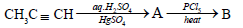The product (A) and (B) are, respectively
Solution:
QUESTION: 5
Which of the following does not produce a white precipitate of AgCl on warming with alcoholic silver nitrate?
Solution:
QUESTION: 6
Which of the following does not repsond positively to the iodoform test ?
Solution:
QUESTION: 7

Which of the following intermediates is formed in the reaction shown below ?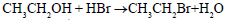Solution:
QUESTION: 8
Which of the following reagents will not convert ethyl alcohol into ethyl chloride ?
Solution:
QUESTION: 9
In the reaction,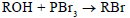the number of moles of alocohol that may be converted into alkyl bromide by one mole of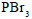is
Solution:
QUESTION: 10
Which of the following compounds is used as a refrigerant
Solution:
QUESTION: 11
Butanenitrile may be prepared by heating
Solution:
QUESTION: 12
In the chlorination of benzene with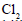in the presence of anhydrous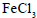,the electrophilic species that attacks the benzene ring is
Solution:
QUESTION: 13
Propene on reaction with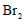in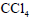yields
Solution:
QUESTION: 14

The volume occupied by 0.5 mole of carbon dioxide at a pressure of 150kPa and at the temperature of 19 °C will be

Solution:
QUESTION: 15
Propene on reaction with ICl produces mainly
Solution:
QUESTION: 16
The dimensions of the coefficient of visocity are
Solution:
QUESTION: 17
The viscous force on a small sphere of radius R moving in a fluid varies with
Solution:
QUESTION: 18
Heating effect is oberserved in a gas when in is allowed to expand because
Solution:
QUESTION: 19
Ozonized oxygen contains 80%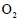by volume. The weight of 1 L of this mixture at stp is approximately
Solution:
QUESTION: 20
The time taken for effusion of 32 mL ofwill be the same as that taken by
Solution:
*Answer can only contain numeric values
QUESTION: 21

A sample of an ideal gas is expanded from 1m3 to 3m3 in a reversible process for which P = KV2, with K = 6 bar/m6. What is work done by the gas (in kJ)?

Solution: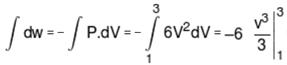= –2 (33 – 13) = – 52 bar.m3 = – 5200 kJ
so, work done by the gas is 5200 kJ

*Answer can only contain numeric values
QUESTION: 22

How many different dichlorides, including stereoisomers by Wurtz coupling reaction with ethereal solution of sodium, can give 1,4-dimethyl cyclohexane ?

Solution:

In 1-4-dimethyl cyclohexane, the following indicated bonds can be formed in the given reaction condition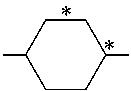Therefore following dichlorides can be used for this purpose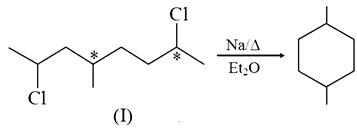(I) has two chiral carbons, hence it has four stereoisomers.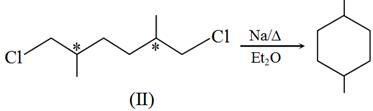(II) Also has two chiral carbons but there are only three stereoismers as one of them is meso.

*Answer can only contain numeric values
QUESTION: 23

When ortho dibromobenzene is subjected to mononitration X number of product are formed and when meta dibromobenzene is subjected to mononitration, Y number of products are formed. Report your answer as XY

Solution:
*Answer can only contain numeric values
QUESTION: 24

A mixture containing all the stereoisomers of 3, 4, 5-trimethyl cyclopentene is treated with O3 followed by Zn-hydrolysis, how many different isomers of products would result?

Solution: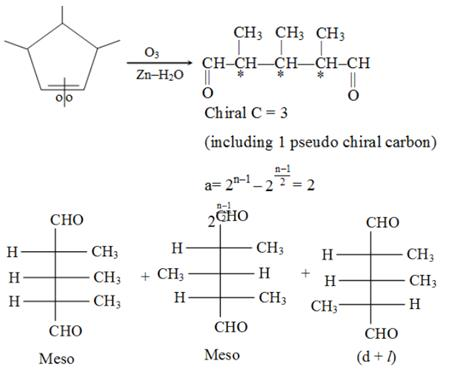*Answer can only contain numeric values
QUESTION: 25

How many of following are soluble in NaHCO3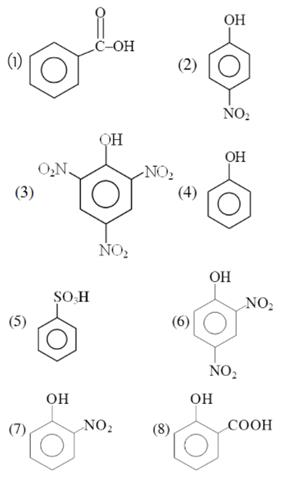Solution:

(1), (2), (3), (5), (6), (8) are soluble in NaHCO3